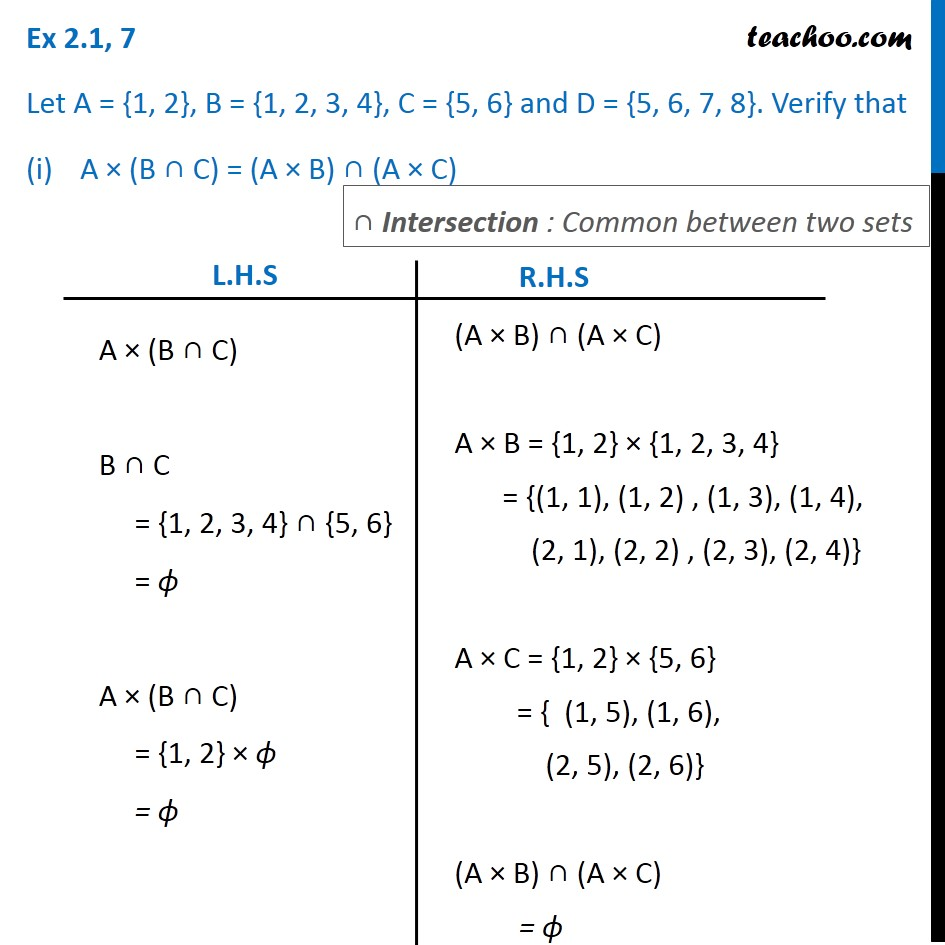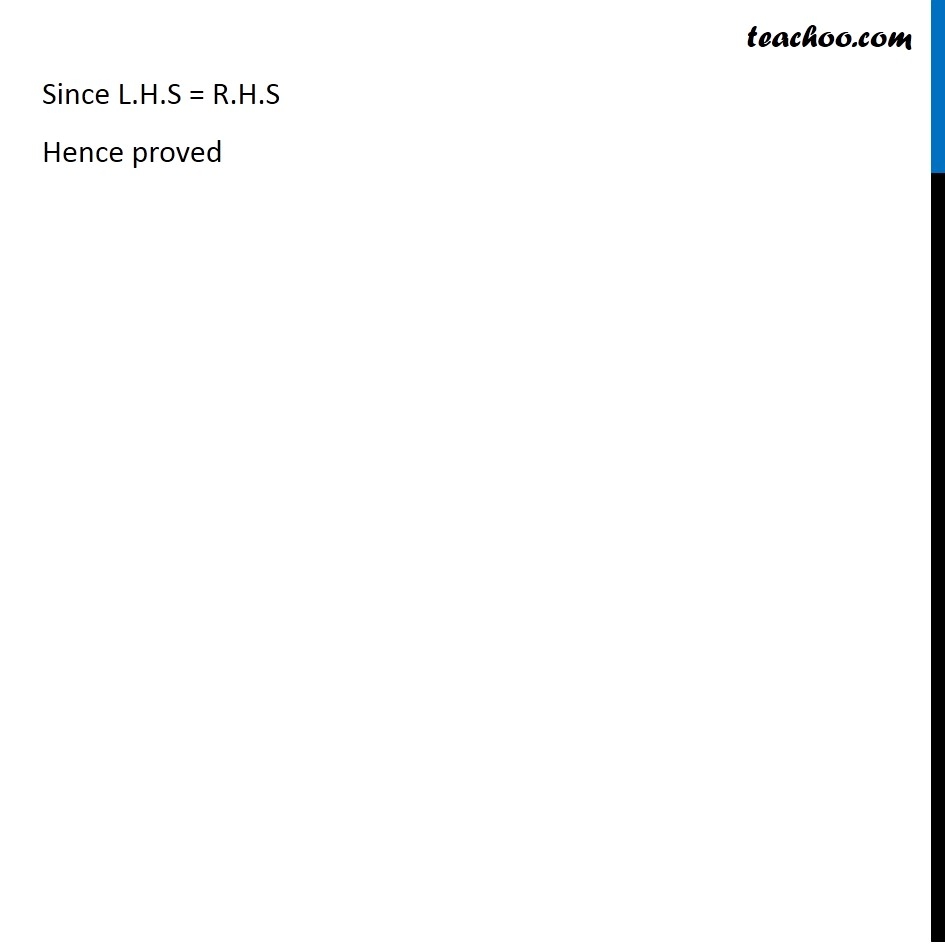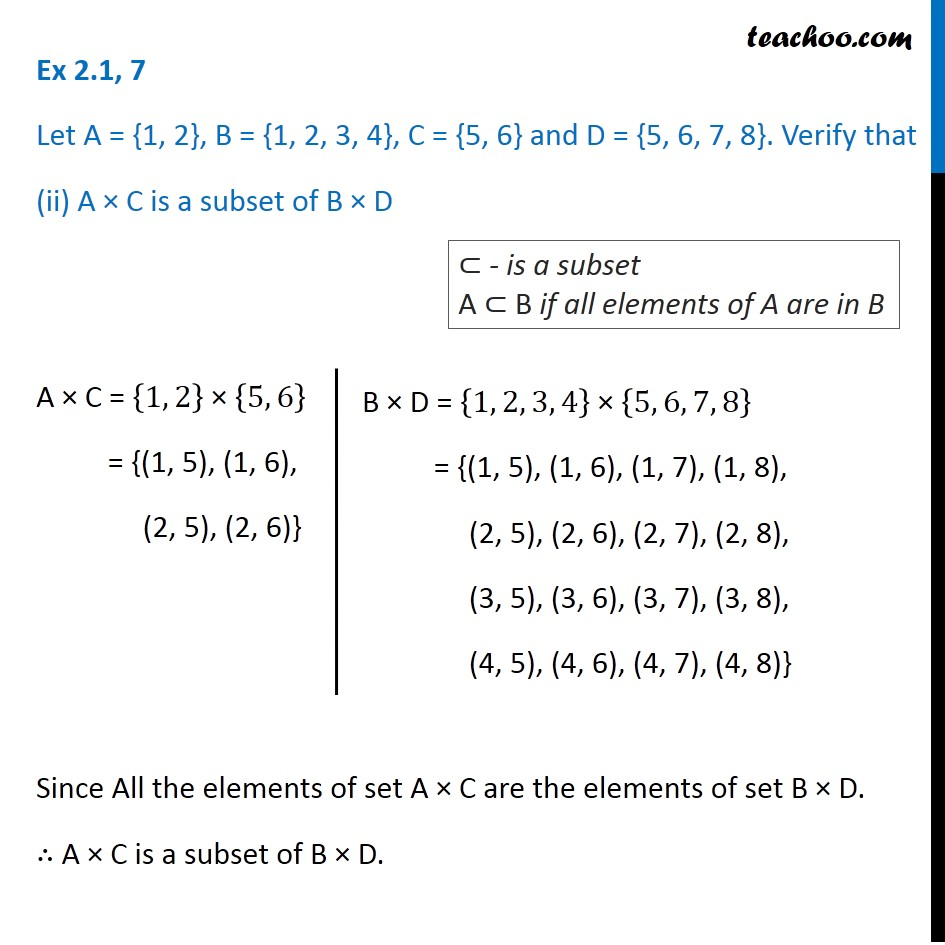Learn All Concepts of Chapter 2 Class 11 Relations and Function - FREE. Check - Relation and Function Class 11 - All Concepts1. Chapter 2 Class 11 Relations and Functions
2. Serial order wise
3. Ex 2.1

Transcript

Ex 2.1, 7 Let A = {1, 2}, B = {1, 2, 3, 4}, C = {5, 6} and D = {5, 6, 7, 8}. Verify that A × (B ∩ C) = (A × B) ∩ (A × C) ∩ Intersection : Common between two sets A × (B ∩ C) B ∩ C = {1, 2, 3, 4} ∩ {5, 6} = ϕ A × (B ∩ C) = {1, 2} × ϕ = ϕ (A × B) ∩ (A × C) A × B = {1, 2} × {1, 2, 3, 4} = {(1, 1), (1, 2) , (1, 3), (1, 4), (2, 1), (2, 2) , (2, 3), (2, 4)} A × C = {1, 2} × {5, 6} = { (1, 5), (1, 6), (2, 5), (2, 6)} (A × B) ∩ (A × C) = ϕ Since L.H.S = R.H.S Hence proved Ex 2.1, 7 Let A = {1, 2}, B = {1, 2, 3, 4}, C = {5, 6} and D = {5, 6, 7, 8}. Verify that (ii) A × C is a subset of B × D A × C = {1, 2} × {5, 6} = {(1, 5), (1, 6), (2, 5), (2, 6)} Since All the elements of set A × C are the elements of set B × D. ∴ A × C is a subset of B × D. ⊂ - is a subset A ⊂ B if all elements of A are in B B × D = {1, 2, 3, 4} × {5, 6, 7, 8} = {(1, 5), (1, 6), (1, 7), (1, 8), (2, 5), (2, 6), (2, 7), (2, 8), (3, 5), (3, 6), (3, 7), (3, 8), (4, 5), (4, 6), (4, 7), (4, 8)}

Ex 2.1